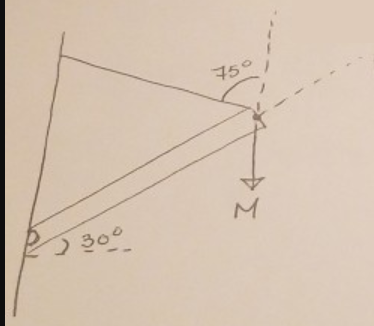# Problem: A beam of mass 100 kg is attached to the wall and allowed to pivot. A cable is attached to the other end of the beam to prevent the beam from pivoting. At the pivot point, there exists support forces Sx &amp; Sy. If the beam is 10 m and an additional mass M is hung from the end of the beam; compute the largest mass that can be hung if the cable has a load limit of 15000 N.

###### FREE Expert Solution

At equilibrium, net torque is zero.

$\overline{)\mathbf{\sum }_{\mathbf{i}}{{\mathbf{\tau }}}_{{\mathbf{i}}}{\mathbf{=}}{\mathbf{0}}}$

84% (284 ratings)###### Problem Details

A beam of mass 100 kg is attached to the wall and allowed to pivot. A cable is attached to the other end of the beam to prevent the beam from pivoting. At the pivot point, there exists support forces Sx & Sy. If the beam is 10 m and an additional mass M is hung from the end of the beam; compute the largest mass that can be hung if the cable has a load limit of 15000 N.